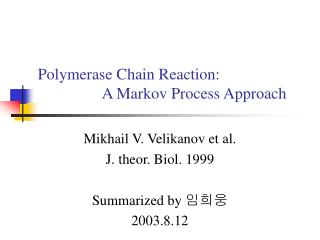DownloadDownload PresentationPolymerase Chain Reaction: A Markov Process Approach

# Polymerase Chain Reaction: A Markov Process Approach

Télécharger la présentation## Polymerase Chain Reaction: A Markov Process Approach

- - - - - - - - - - - - - - - - - - - - - - - - - - - E N D - - - - - - - - - - - - - - - - - - - - - - - - - - -
##### Presentation Transcript

1. Polymerase Chain Reaction: A Markov Process Approach Mikhail V. Velikanov et al. J. theor. Biol. 1999 Summarized by 임희웅 2003.8.12

2. Contents • Introduction • Markov Process Model for Primer Extension • Model for Multi-Cycle PCR Runs • Numerical Results • Discussion

3. Introduction • A stochastic approach to PCR • Focus on the microscopic nature of amplification process • Elementary reaction: binding of dNTP • Markov process  master equation • Analytical solution for the probability distribution of DNA length • Main qualitative feature • Sensitivity of the reaction condition • The amplification plateau effect • Optimal duration of amplification for each cycle

4. l0 l l+1 … L … Markov Process Model for Primer Extension • Amplification process as Markov process • Binding of dNTP occurs randomly, with the probability per unit time determined entirely by the present state of the system. • State: length of primer • Reaction rate: w = k(t) n • n: total number of dNTP in the current system • k(t): the rate coefficient which depends on temperature (time) • l + n = l0+ n0 = m0 constant • n0: initial total number of dNTP

5. Master Equation • The master equation for the primer extension process

6. Analytical Solution

7. Model for Multi-Cycle PCR Runs • Additional feature • Increasing number of DNA molecules • Statistical independence of the extension process • n0: initial number of dNTPs per template strand • np: the number of primers per template strand • Complementarity • Two kinds of strand: +, - • Pi+(l,n): Prob. distribution of + strand in ith cycle

8. Evolution of Probability Distribution • ηn: duration of the extension phase of each cycle The distribution for the first cycle Consumed dNTP in first cycle

9. Numerical Result • Simulation for PCR Runs

10. Optimization of PCR Runs Arrhenius’ law

11. Discussion • Primary assumption • DNA synthesis occurs independently on each template strand. • Advantage in Markov process approach • The model can be solved exactly by analytical means.  simple calculation • It accounts for the fluctuations inherent in PCR kinetics through a description of their natural microscopic source. • The model is easy to modify and can be used as the basis for constructing dedicated algorithms for numerical simulations of PCR.